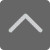## 爱智康资讯站

### 课程咨询: 4000-121-121

• 全国课程在线咨询
• 咨询热线：4000-121-121
• 登录手机官网

• 关注微信公众号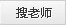# 高一上学期期中考后，函数知识点总结

2020-11-02 16:49:29 　来源：西安爱智康

## 学而思・爱智康1对1/8人班 帮助孩子解决知识难点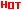*2470人已预约，首课体验不满意，可全额退费。

― ― 报名课程可获得 ― ―

(一)、映射、函数、反函数

1、对应、映射、函数三个概念既有共性又有区别，映射是一种特殊的对应，而函数又是一种特殊的映射.

2、对于函数的概念，应注意如下几点：

(1)掌握构成函数的三要素，会判断两个函数是否为同一函数.

(2)掌握三种表示法——列表法、解析法、图象法，能根实际问题寻求变量间的函数关系式，特别是会求分段函数的解析式.

(3)如果y=f(u)，u=g(x)，那么y=f[g(x)]叫做f和g的复合函数，其中g(x)为内函数，f(u)为外函数.

3、求函数y=f(x)的反函数的一般步骤：

(1)确定原函数的值域，也就是反函数的定义域;

(2)由y=f(x)的解析式求出x=f-1(y);

(3)将x，y对换，得反函数的习惯表达式y=f-1(x)，并注明定义域.

注意①：对于分段函数的反函数，先分别求出在各段上的反函数，然后再合并到一起.

②熟悉的应用，求f-1(x0)的值，合理利用这个结论，可以避免求反函数的过程，从而简化运算.

(二)、函数的解析式与定义域

1、函数及其定义域是不可分割的整体，没有定义域的函数是不存在的，因此，要正确地写出函数的解析式，必须是在求出变量间的对应法则的同时，求出函数的定义域.求函数的定义域一般有三种类型：

(1)有时一个函数来自于一个实际问题，这时自变量x有实际意义，求定义域要结合实际意义考虑;

(2)已知一个函数的解析式求其定义域，只要使解析式有意义即可.如：

①分式的分母不得为零;

②偶次方根的被开方数不小于零;

③对数函数的真数必须大于零;

④指数函数和对数函数的底数必须大于零且不等于1;

⑤三角函数中的正切函数y=tanx(x∈R，且k∈Z)，余切函数y=cotx(x∈R，x≠kπ，k∈Z)等.

应注意，一个函数的解析式由几部分组成时，定义域为各部分有意义的自变量取值的公共部分(即交集).

(3)已知一个函数的定义域，求另一个函数的定义域，主要考虑定义域的深刻含义即可.

已知f(x)的定义域是[a，b]，求f[g(x)]的定义域是指满足a≤g(x)≤b的x的取值范围，而已知f[g(x)]的定义域[a，b]指的是x∈[a，b]，此时f(x)的定义域，即g(x)的值域.

2、求函数的解析式一般有四种情况

(1)根据某实际问题需建立一种函数关系时，必须引入合适的变量，根据数学的有关知识寻求函数的解析式.

(2)有时题设给出函数特征，求函数的解析式，可采用待定系数法.比如函数是一次函数，可设f(x)=ax+b(a≠0)，其中a，b为待定系数，根据题设条件，列出方程组，求出a，b即可.

(3)若题设给出复合函数f[g(x)]的表达式时，可用换元法求函数f(x)的表达式，这时必须求出g(x)的值域，这相当于求函数的定义域.

(4)若已知f(x)满足某个等式，这个等式除f(x)是未知量外，还出现其他未知量(如f(-x)，等)，必须根据已知等式，再构造其他等式组成方程组，利用解方程组法求出f(x)的表达式.

(三)、函数的值域与最值

1、函数的值域取决于定义域和对应法则，不论采用何种方法求函数值域都应先考虑其定义域，求函数值域常用方法如下：

(1)直接法：亦称观察法，对于结构较为简单的函数，可由函数的解析式应用不等式的性质，直接观察得出函数的值域.

(2)换元法：运用代数式或三角换元将所给的复杂函数转化成另一种简单函数再求值域，若函数解析式中含有根式，当根式里一次式时用代数换元，当根式里是二次式时，用三角换元.

(3)反函数法：利用函数f(x)与其反函数f-1(x)的定义域和值域间的关系，通过求反函数的定义域而得到原函数的值域，形如(a≠0)的函数值域可采用此法求得.

(4)配方法：对于二次函数或二次函数有关的函数的值域问题可考虑用配方法.

(5)不等式法求值域：利用基本不等式a+b≥[a，b∈(0，+∞)]可以求某些函数的值域，不过应注意条件“一正二定三相等”有时需用到平方等技巧.

(6)判别式法：把y=f(x)变形为关于x的一元二次方程，利用“△≥0”求值域.其题型特征是解析式中含有根式或分式.

(7)利用函数的单调性求值域：当能确定函数在其定义域上(或某个定义域的子集上)的单调性，可采用单调性法求出函数的值域.

(8)数形结合法求函数的值域：利用函数所表示的几何意义，借助于几何方法或图象，求出函数的值域，即以数形结合求函数的值域.

2、求函数的最值与值域的区别和联系

求函数最值的常用方法和求函数值域的方法基本上是相同的，事实上，如果在函数的值域中存在一个最小(大)数，这个数就是函数的最小(大)值.因此求函数的最值与值域，其实质是相同的，只是提问的角度不同，因而答题的方式就有所相异.

如函数的值域是(0，16]，最大值是16，无最小值.再如函数的值域是(-∞，-2]∪[2，+∞)，但此函数无最大值和最小值，只有在改变函数定义域后，如x>0时，函数的最小值为2.可见定义域对函数的值域或最值的影响.

3、函数的最值在实际问题中的应用

函数的最值的应用主要体现在用函数知识求解实际问题上，从文字表述上常常表现为“工程造价最低”，“利润最大”或“面积(体积)最大(最小)”等诸多现实问题上，求解时要特别关注实际意义对自变量的制约，以便能正确求得最值.

(四)、函数的奇偶性

1、函数的奇偶性的定义：对于函数f(x)，如果对于函数定义域内的任意一个x，都有f(-x)=-f(x)(或f(-x)=f(x))，那么函数f(x)就叫做奇函数(或偶函数).

正确理解奇函数和偶函数的定义，要注意两点：(1)定义域在数轴上关于原点对称是函数f(x)为奇函数或偶函数的必要不充分条件;(2)f(x)=-f(x)或f(-x)=f(x)是定义域上的恒等式.(奇偶性是函数定义域上的整体性质).

2、奇偶函数的定义是判断函数奇偶性的主要依据。为了便于判断函数的奇偶性，有时需要将函数化简或应用定义的等价形式：

注意如下结论的运用：

(1)不论f(x)是奇函数还是偶函数，f(|x|)总是偶函数;

(2)f(x)、g(x)分别是定义域D1、D2上的奇函数，那么在D1∩D2上，f(x)+g(x)是奇函数，f(x)·g(x)是偶函数，类似地有“奇±奇=奇”“奇×奇=偶”，“偶±偶=偶”“偶×偶=偶”“奇×偶=奇”;

(3)奇偶函数的复合函数的奇偶性通常是偶函数;

(4)奇函数的导函数是偶函数，偶函数的导函数是奇函数。

3、有关奇偶性的几个性质及结论

(1)一个函数为奇函数的充要条件是它的图象关于原点对称;一个函数为偶函数的充要条件是它的图象关于y轴对称.

(2)如要函数的定义域关于原点对称且函数值恒为零，那么它既是奇函数又是偶函数.

(3)若奇函数f(x)在x=0处有意义，则f(0)=0成立.

(4)若f(x)是具有奇偶性的区间单调函数，则奇(偶)函数在正负对称区间上的单调性是相同(反)的。

(5)若f(x)的定义域关于原点对称，则F(x)=f(x)+f(-x)是偶函数，G(x)=f(x)-f(-x)是奇函数.

(6)奇偶性的推广

函数y=f(x)对定义域内的任一x都有f(a+x)=f(a-x)，则y=f(x)的图象关于直线x=a对称，即y=f(a+x)为偶函数.函数y=f(x)对定义域内的任-x都有f(a+x)=-f(a-x)，则y=f(x)的图象关于点(a，0)成中心对称图形，即y=f(a+x)为奇函数.

(五)、函数的单调性

1、单调函数

对于函数f(x)定义在某区间[a，b]上任意两点x1，x2，当x1>x2时，都有不等式f(x1)>(或<)f(x2)成立，称f(x)在[a，b]上单调递增(或递减);增函数或减函数统称为单调函数.

对于函数单调性的定义的理解，要注意以下三点：

(1)单调性是与“区间”紧密相关的概念.一个函数在不同的区间上可以有不同的单调性.

(2)单调性是函数在某一区间上的“整体”性质，因此定义中的x1，x2具有任意性，不能用特殊值代替.

(3)单调区间是定义域的子集，讨论单调性必须在定义域范围内.

(4)注意定义的两种等价形式：

设x1、x2∈[a，b]，那么：

①在[a、b]上是增函数;

在[a、b]上是减函数.

②在[a、b]上是增函数.

在[a、b]上是减函数.

需要指出的是：①的几何意义是：增(减)函数图象上任意两点(x1，f(x1))、(x2，f(x2))连线的斜率都大于(或小于)零.

(5)由于定义都是充要性命题，因此由f(x)是增(减)函数，且(或x1>x2)，这说明单调性使得自变量间的不等关系和函数值之间的不等关系可以“正逆互推”.

5、复合函数y=f[g(x)]的单调性

若u=g(x)在区间[a，b]上的单调性，与y=f(u)在[g(a)，g(b)](或g(b)，g(a))上的单调性相同，则复合函数y=f[g(x)]在[a，b]上单调递增;否则，单调递减.简称“同增、异减”.

在研究函数的单调性时，常需要先将函数化简，转化为讨论一些熟知函数的单调性。因此，掌握并熟记一次函数、二次函数、指数函数、对数函数的单调性，将大大缩短我们的判断过程.

6、证明函数的单调性的方法

(1)依定义进行证明.其步骤为：①任取x1、x2∈M且x1(或<)f(x2);③根据定义，得出结论.

(2)设函数y=f(x)在某区间内可导.

如果f′(x)>0，则f(x)为增函数;如果f′(x)<0，则f(x)为减函数.

(六)、函数的图象

函数的图象是函数的直观体现，应加强对作图、识图、用图能力的培养，培养用数形结合的思想方法解决问题的意识.

求作图象的函数表达式

与f(x)的关系

由f(x)的图象需经过的变换

y=f(x)±b(b>0)

沿y轴向平移b个单位

y=f(x±a)(a>0)

沿x轴向平移a个单位

y=-f(x)

作关于x轴的对称图形

y=f(|x|)

右不动、左右关于y轴对称

y=|f(x)|

上不动、下沿x轴翻折

y=f-1(x)

作关于直线y=x的对称图形

y=f(ax)(a>0)

横坐标缩短到原来的，纵坐标不变

y=af(x)

纵坐标伸长到原来的|a|倍，横坐标不变

y=f(-x)

作关于y轴对称的图形

【例】定义在实数集上的函数f(x)，对任意x，y∈R，有f(x+y)+f(x-y)=2f(x)·f(y)，且f(0)≠0.

①求证：f(0)=1;

②求证：y=f(x)是偶函数;

③若存在常数c，使求证对任意x∈R，有f(x+c)=-f(x)成立;试问函数f(x)是不是周期函数，如果是，找出它的一个周期;如果不是，请说明理由.

思路分析：我们把没有给出解析式的函数称之为抽象函数，解决这类问题一般采用赋值法.

解答：①令x=y=0，则有2f(0)=2f2(0)，因为f(0)≠0，所以f(0)=1.

②令x=0，则有f(x)+f(-y)=2f(0)·f(y)=2f(y)，所以f(-y)=f(y)，这说明f(x)为偶函数.

③分别用(c>0)替换x、y，有f(x+c)+f(x)=

所以，所以f(x+c)=-f(x).

两边应用中的结论，得f(x+2c)=-f(x+c)=-[-f(x)]=f(x)，

所以f(x)是周期函数，2c就是它的一个周期.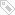标签：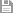保存　｜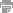打印　｜关闭